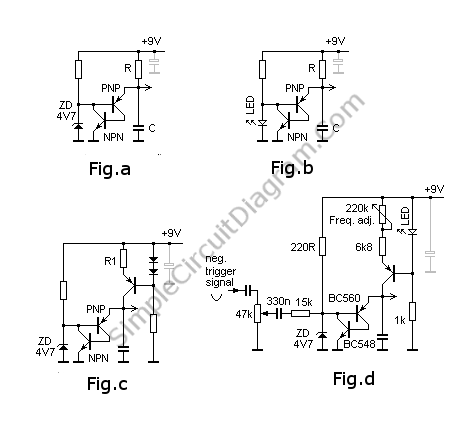# Sawtooth Generator (Oscillator) with Transistors

In the figure a, the capacitor is slowly charged through resistor R and finally after reaching the base-emitter voltage of PNP transistor, the PNP transistor begin conducting and activate the NPN transistor. The activation of NPN transistor is positively fed back to the PNP transistor so the transistors short the capacitor C to ground, discharging its voltage until zero in very fast manner. After the capacitor voltage falls down to zero, the transistors are turned off again, and the cycle of charging the cap C through resistor R is repeated.The zener diode voltage determine the level when the cap discharge should occurs, when this voltage is too high, then the curve of cap voltage will be logarithmic, not expected as sawtooth which is linear. By keeping this voltage level far below the battery source (9V), an approximation of linear curve is produced, enough for many simple applications. Figure b replace the zener diode with a LED, one kind of trick to get a lower discharge level if can’t find lower voltage zener diode.

A true linear curve can be produced if we replace the resistor R with a constant current source, as shown in the schematic diagram in figure c. A simple triggering mechanism can be obtained by circuit modification as shown in the schematic diagram figure d. [Circuit’s schematic diagram source: Claude Jacobs]

Basic circuit:
In Abb.1a wird zun